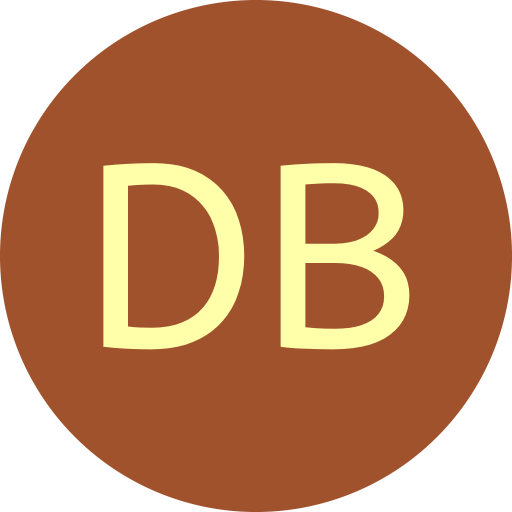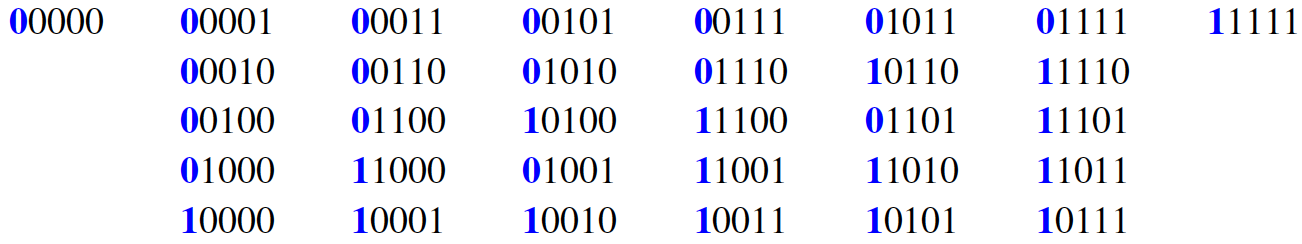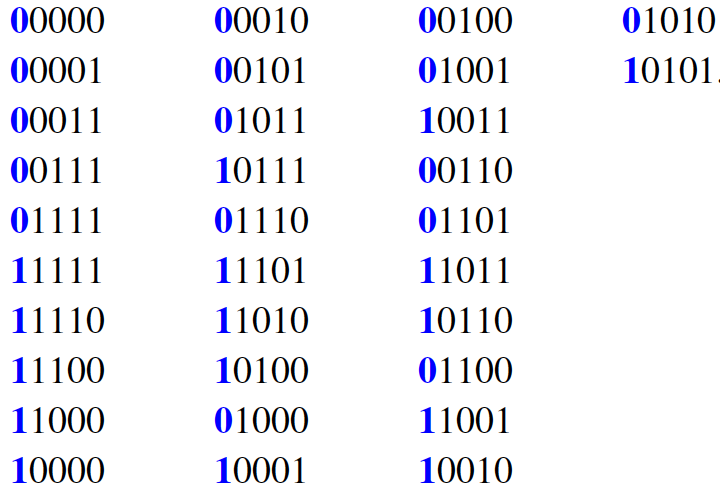#De Bruijn Sequence and Universal Cycle Constructions

Concatentation Method Output
 Algorithm Granddaddy Grandmama Lex compositions Generic: f = r(a₁) + s Cool-lex Order $n$ Symbols $k$ 2 3 4 5 6 7 8 9
Constructing DB sequences using concatenation approaches

Concatenation constructions [FM78,DHS+18,GS17,GS18] have led to the most efficient algorithms to construct DB sequences, with some obtaining $O(1)$-amortized time per symbol. These running times are realized for both the Granddaddy (lex smallest DB sequence) and the Grandmama, which also have $O(n)$-time shift rules per symbol.

#### Necklace concatenations and the PCR

A necklace is the lexicographically smallest string in an equivalence class under rotation. For instance, such equivalence classes for $n=5$ and $k=2$ are given below, where the top string in each class is a necklace. The classes are listed in lex order with respect to their necklace representative.The longest aperiodic prefix of each necklace corresponds to the cycle induced by the Pure Cycling Register (PCR). In the above example, the longest aperiodic prefix of each necklace corresponds to the blue bits in each column read top to bottom. The Granddaddy (lex smallest) DB sequence is constructed as follows [FM78] for a given $n,k$:

1. List the necklaces of length $n$ with alphabet size $k$ in lex order.
2. Concatenate together the longest aperiodic prefixes of each necklace.
For example, for $n=5$ and $k=2$ we obtain the DB sequence:

0 00001 00011 00101 00111 01011 01111 1.

Since necklaces can be generated in lex order in $O(1)$ amortized time [RSW92], the DB sequence can be constructed in $O(1)$ amortized time per each $n$-symbols generated. The Granddaddy sequence can also be constructed by the greedy Prefer-largest construction and the shift rule PCR1. Amazingly, it took almost 40 years to realize that by replacing lex order with colex order, the concatenation approach will again yield a DB sequence [DHW16,DHW+18].

Grandmama Concatenation Construction
1. List the necklaces of length $n$ with alphabet size $k$ in colex order.
2. Concatenate together the longest aperiodic prefixes of each necklace.
For $n=5$ and $k=2$, we obtain:

0 00001 00101 00011 01011 00111 01111 1.

A reason for this late discovery is that the concatenation method for lex order has frequently been described using Lyndon words, which are primitive (aperiodic) necklaces. In particular the Granddaddy corresponds to concatenating all the Lyndon words with length that divides $n$ in lex order. This construction, however, does not generalize to colex order. The Grandmama sequence can also be constructed via the shift rule PCR2.

In addition to these three concatenation constructions, there is a third based on the PCR and two more based on the CCR [GS18]. One of the CCR constructions is outlined next.

#### A generic approach and the CCR

The above concatenation strategies can be stated more generally for an arbitrary non-singular feedback function $f$.

Generic Concatenation Approach
1. Order the equivalence classes induced by the feedback function $f$.
2. Concatenate together defined representatives for each cycle of an equivalence class.
Applying this approach in the binary case, using the Complementing Cycling Register (CCR) for $f$, we obtain the following equivalence classes ordered in lex order with respect to their lex smallest element.If the cycles are represented by their lex smallest rotation (the blue columns read top to bottom), then the concatenation approach yields the following sequence:

0000011111 0001011101 0010011011 01.

Since the substring 11010 appears twice (once in wraparound), this is not a DB sequence. However, if the classes are re-ordered using colex order of their lex smallest element at the top of each column, a DB sequence is obtained [GS17,GS18]:

0000011111 0010011011 0001011101 01.

This resulting DB sequence based on the CCR can also be constructed via the shift rule CCR2.

More generally, if a feedback function is of the form $f(a_1a_2\cdots a_n) = ra_1 + s$ and using colex order to order the smaller cycles, then the concatenation approach will produce a DB sequence using the lex smallest cycle representatives if:

1. $1 \leq r \leq k{-}1$ and $r$ is coprime with $k$, and
2. $0 \leq s \leq k{-}1$ and ($r=1$ or $s$ is coprime with $k$)

#### Lexicographic compositions

An early attempt to find an efficient shift-rule for the binary Prefer-same greedy construction resulted in a concatenation construction based on lexicographic compositions [FK77]. The algorithm description is rather complex; however, there is a simpler shift rule interpretation based on the underlying feedback function $f(a_1a_2\cdots a_n) = a_1 + a_2 + a_n$ [SSA20].

#### Cool-lex order

Using cool-lex order for binary strings, an underlying concatenation scheme is used to construct a dual-density DB sequence for bit strings with density (weight) $d$ and $d+1$ [RSW12]. A simple strategy for joining these cycles together produces a density-range DB sequence from 0 to $d$. When $d=n$, a DB sequence is produced and can be generated above. The C program available for download can produce both sequences, along with listings of necklaces and Lyndon words in cool-lex Gray code order.

References

• [FK77] H. Fredricksen and I. Kessler. Lexicographic compositions and deBruijn sequences. J. Combinatorial Theory Ser. A, 22(1):17-30, 1977.

• [FM78] H. Fredricksen and J. Maiorana. Necklaces of beads in $k$ colors and $k$-ary de Bruijn sequences. Discrete Math., 23(3):207-210, 1978.

• [DHS+18] P. B. Dragon, O. I. Hernandez, J. Sawada, A. Williams, and D. Wong. Constructing de Bruijn sequences with co-lexicographic order: the $k$-ary Grandmama sequence. European J. Combin., 72:1-11, 2018.

• [DHW16] P. B. Dragon, O. I. Hernandez, and A. Williams. The grandmama de Bruijn sequence for binary strings. In LATIN 2016: theoretical informatics, volume 9644 of Lecture Notes in Comput. Sci., pages 347-361. Springer, Berlin, 2016.

• [GS17] D. Gabric and J. Sawada. A de Bruijn sequence construction by concatenating cycles of the complemented cycling register. In Combinatorics on words, volume 10432 of Lecture Notes in Comput. Sci., pages 49-58. Springer, Cham, 2017.

• [GS18] D. Gabric and J. Sawada. Constructing de Bruijn sequences by concatenating smaller universal cycles. Theoret. Comput. Sci., 743:12-22, 2018.

• [RSW92] F. Ruskey, C. Savage, and T. M. Y. Wang. Generating necklaces. J. Algorithms, 13(3):414-430, 1992.

• [RSW12] F. Ruskey, J. Sawada, and A. Williams. De Bruijn sequences for fixed-weight binary strings. SIAM J. Discrete Math., 26(2):605-617, 2012.

• [SSA20] E. Sala, J. Sawada, A. Alhakim. Efficiently constructing the Prefer same de Bruijn sequence. Submitted 2020.